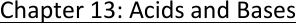Textbook Notes (270,000)
US (100,000)
U of M (2,000)
CHEM (100)
CHEM 130 (100)
Chapter 13

CHEM 130 Chapter Notes - Chapter 13: Conjugate Acid, Equilibrium Constant, Acid Strength

Department
Chemistry
Course Code
CHEM 130
Professor
Carol Ann Castaneda
Chapter
13

This preview shows page 1. to view the full 5 pages of the document.Chapter 13: Acids and Bases
13.1: The Nature of Acids and Bases
o Acids were first recognized as a class of substances that taste sour
o Bases, sometimes called alkalis, are characterized by their bitter taste and slippery feel
o Arrhenius postulated that acids produce hydrogen ions in aqueous solution, while bases
produce hydroxide ions
o Arrhenius concept is limited because it applies only to aqueous solutions and allows for only
one kind of base - the hydroxide ion
o The Bronsted-Lowry model, an acid is a proton (H+) donor, and a base is a proton acceptor
o Hydronium ion: the H3O+; a hydrated ion
o Water is amphoteric; can act as an acid or a base
o The conjugate base is everything that remains of the acid molecule after a proton is lost
o The conjugate acid is formed when the proton is transferred to the base
o A conjugate acid-base pair consists of two substances related to each other by donating and
accepting of a single proton
o A competition for the proton between the two bases H2O and A-
o If H2O is a much stronger base than A-, that is if H2O has a much greater affinity for H+ than
does A-, the equilibrium position will be far to the right
Most of the acid dissolved will be in the ionized form
o If A- is a much stronger base than H2O, the equilibrium position will lie far to the left
Most of the acid dissolved will be present at equilibrium as HA
o Acid disassociation constant: The equilibrium constant for a reaction in which a proton is
removed from an acid by H2O to form the conjugate base and H3O+
o Ka is the equilibrium constant for the reaction in which a proton is removed from HA to form
the conjugate base A-
o The Bronsted-Lowry model is not limited to aqueous solutions; it can be extended to reactions
in the gas phase
13.2: Acid strength
o A strong acid is one for which this equilibrium lies far to the right
o Almost all the original HA is disassociated (ionized) at equilibrium
o A strong acid yields a weak conjugate base - one that las a low affinity for a proton
o A strong acid also can be described as an acid whose conjugate base is a much weaker base
than water
o In this case the water molecules win the competition for the H+ ions
o A weak acid HB consists of mostly undissociated HB molecules in water
o A weak acid is one for which the equilibrium lies far to the left
o Most of the acid originally placed in the solution is still present as HA at equilibrium
o In contrast to a strong acid, a weak acid has a conjugate base that is a much stronger base
than water
o In this case a water molecule is not very successful in pulling an H+ ion from the conjugate
base
o The weaker the acid, the stronger its conjugate base
o The common strong acids are:
Sulfuric acid (H2SO4)
Hydrochloric acid (HCl)
Nitric acid (HNO3)

Unlock to view full version

Only page 1 are available for preview. Some parts have been intentionally blurred.

Perchloric acid (HClO4)
o Sulfuric acid is a diprotic acid, an acid having two acidic protons
o Many acids are oxyacids, in which the acidic proton is attached to an oxygen atom
o Many common weak acids (oxyacids):
Phosphoric acid (H3PO4)
Nitrous acid (HNO2)
Hypochlorous acid (HOCl)
o Organic acids, those with a carbon atom backbone, commonly contain a carboxyl group
Examples:
Acetic acid (CH3COOH)
Benzoic acid (C6H5COOH)
o Acids of this type are usually weak
o There are some important acids in which the acidic proton is attached to an atom other an
oxygen
o The most significant of these are the hydrohalic acids HX, where X represents a halogen atom
o List of common monoprotic acids:
Hydrogen sulfate ion
Chlorous acid
Monochloroacetic acid
Hydrofluoric acid
Nitrous acid
Acetic acid
Hydrated aluminum (III) ion
Hypochlorous acid
Hydrocyanic acid
Ammonium ion
Phenol
o Water as an acid and a base:
A substance is said to be amphoteric if it can behave wither as an acid or as a base
Water is the most common amphoteric substance
Autoionization of water, which involved the transfer of a proton from one water
molecule to another to produce a hydroxide ion and a hydronium ion
Kw, called the ion-product constant (or the dissociation constant for water), always
refers to the autoionization of water
Since Kw is an equilibrium constant, it varies with temperature
13.3: The pH Scale
o The pH scale provides a convenient way to represent solution acidity
o The number of decimal places in the log is equal to the number of significant figures in the
original number
o Since pH is a log scale based on 10, the pH changes by 1 for every power of 10 change in H+
o PH is defined as -log[H+]
o The pH decreases as [H+] increases
o The pH of a solution is usually measured using a pH meter, an electronic device with a probe
that can be inserted into a solution of unknown pH
o The probe contains an acidic aqueous solution enclosed by a special glass membrane that
allows migration of H+ ions
o If the unknown solution has a different pH from the solution in the probe, the electric
potential results, which is registered on the meter